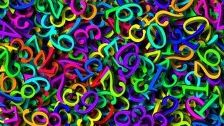# Some Problems in Number TheoryNumber theory - the study of the natural numbers - does not typically feature in school curricula, but it is a rich source of interesting problems which can lead to surprising results. The problems in this feature will offer you the opportunity to notice patterns, conjecture, generalise and prove results.

Many results can be explained by applying GCSE algebraic techniques, and many can also be explained using diagrams or pictorial representations.

Number theory is studied in more detail at university, and it is often seen as one of the "purest" forms of mathematics.  Gauss said "Mathematics is the queen of the sciences - and number theory is the queen of mathematics".

You can watch a recording of the webinar in which we discussed the mathematical thinking which can be prompted by these problems.

### Always Perfect

##### Age 14 to 18Challenge Level
Show that if you add 1 to the product of four consecutive numbers the answer is ALWAYS a perfect square.

### Common Divisor

##### Age 14 to 18Challenge Level
Can you find out what numbers divide these expressions? Can you prove that they are always divisors?

### Polite Numbers

##### Age 16 to 18Challenge Level
A polite number can be written as the sum of two or more consecutive positive integers, for example 8+9+10=27 is a polite number. Can you find some more polite, and impolite, numbers?

### Digital Equation

##### Age 16 to 18Challenge Level
Can you find a three digit number which is equal to the sum of the hundreds digit, the square of the tens digit and the cube of the units digit?

### Seeing Is Believing

##### Age 11 to 18
In this article for teachers we draw attention to the value of visual representations.

We are very grateful to the Heilbronn Institute for Mathematical Research for their generous support for the development of these resources.